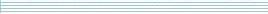| home      | people     | research     | publications    | seminars    | events     | contactCommunication Networks   | Systems Biology   | Hybrid Systems    | Machine Learning   | Dynamics & Interaction### Schools Mathematics Grand Challenge

Week three's Puzzles

Problem 4:

The fourth problem was:

A new hotel has 40 floors, which are numbered from 1 to 40, and a nice new shiny elevator. The elevator travels at a constant speed and takes exactly 7 seconds to go from the floor 1 to floor 6(if it does not stop in between). How long does it take to go from floor 1 to the floor 36 (again if it does not stop in between)?

The solution was:

It takes the lift 7 seconds to go from floor 1 to floor 6. Thus it takes 7 seconds to travel up 6 - 1 = 5 floors.

To go from floor 1 to floor 36, the lift needs to travel up 36 - 1 = 35 floors. The distance from floor 1 to floor 36 is 7 times the distance from floor 1 to floor 6. Thus, it will take 7 x 7 = 49 seconds to travel from floor 1 to floor 36.

Problem 5:

The fifth problem was:

Mary has recently purchased a second-hand car for less than 5000 euro. The garage will allow her to pay for the car in ten different ways. She can pay for the car in one single payment, or in 2, 3, 4, 5, 6, 7, 8, 9 or 10 equal instalments. For example, if the car costs 1,000 euro (which it doesn't!) she could make 1 payment of 1,000 euro, 2 payments of 500 euro, 3 payments of 333.33 euro, 4 payments of 250 euro and so on.

However, Mary has noticed that, whichever payment scheme she chooses, all of the amounts she pays are whole numbers of euro (so a payment of 333.33 can never happen). How much did Mary's car cost?

The solution was:

The price of Mary's car must be a multiple of all of the numbers 2, 3, 4, 5, 6, 7, 8, 9 and 10. The smallest such number is the least common multiple of these:

2 x 2 x 2 x 3 x 3 x 5 x 7 = 2520.

The next smallest number with this property is 5040. But we are told that the car cost less than 5000. Hence, the answer is 2520 euro.

Problem 6:

The sixth problem was:

What is the only two digit number (a number between 10 and 99 inclusive) with the following property?

If you add its digits together and multiply the answer by 6, you recover the original number.

The solution was:

We can write any two-digit number as 10 x a + b where 1 ≤ a ≤ 9 and 0 ≤ b ≤ 9. The number in the problem must satisfy

6 x (a + b) = 10 x a + b

So

5 x b = 4 x a .

This means that 5 must divide into a. So the only possiblity for a is actually 5. But then, b must be 4. So the number is 54.